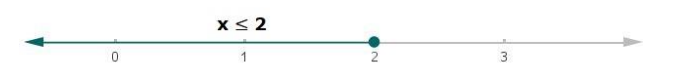# Solve each of the following in equations and represent the solution set on`
Question:

Solve each of the following in equations and represent the solution set on the number line.

$3-2 x \geq 4 x-9$, where $x \in R$

Solution:

Given:

$3-2 x \geq 4 x-9$, where $x \in R$

$3-2 x \geq 4 x-9$

Subtracting 3 from both the sides in the above equation,

$3-2 x-3 \geq 4 x-9-3$

$-2 x \geq 4 x-12$

Now, subtracting 4x from both the sides in the above equation,

$-2 x-4 x \geq 4 x-12-4 x$

$-6 x \geq-12$

Now, dividing both the sides by 6 in the above equation

$\frac{-6 x}{6} \geq \frac{-12}{6}$

$-x \geq-2$

Now, multiplying by $(-1)$ on both the sides in above equation.

$(-x) \cdot(-1) \geq(-2) \cdot(-1)$

$x \leq 2$